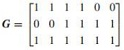Determine the rate-minimum distance and the coding gain

Solve the following problem:

C is a (6, 3) linear block code whose generator matrix is given by1. What rate, minimum distance, and the coding gain can C provide in soft decision decoding when BPSK is used over an AWGN channel?

2. Can you suggest another (6, 3) LBC that can provide a better coding gain? If the answer is yes, what is its generator matrix and the resulting coding gain? If the answer is no, why?

3. Suggest a parity check matrix H for C.